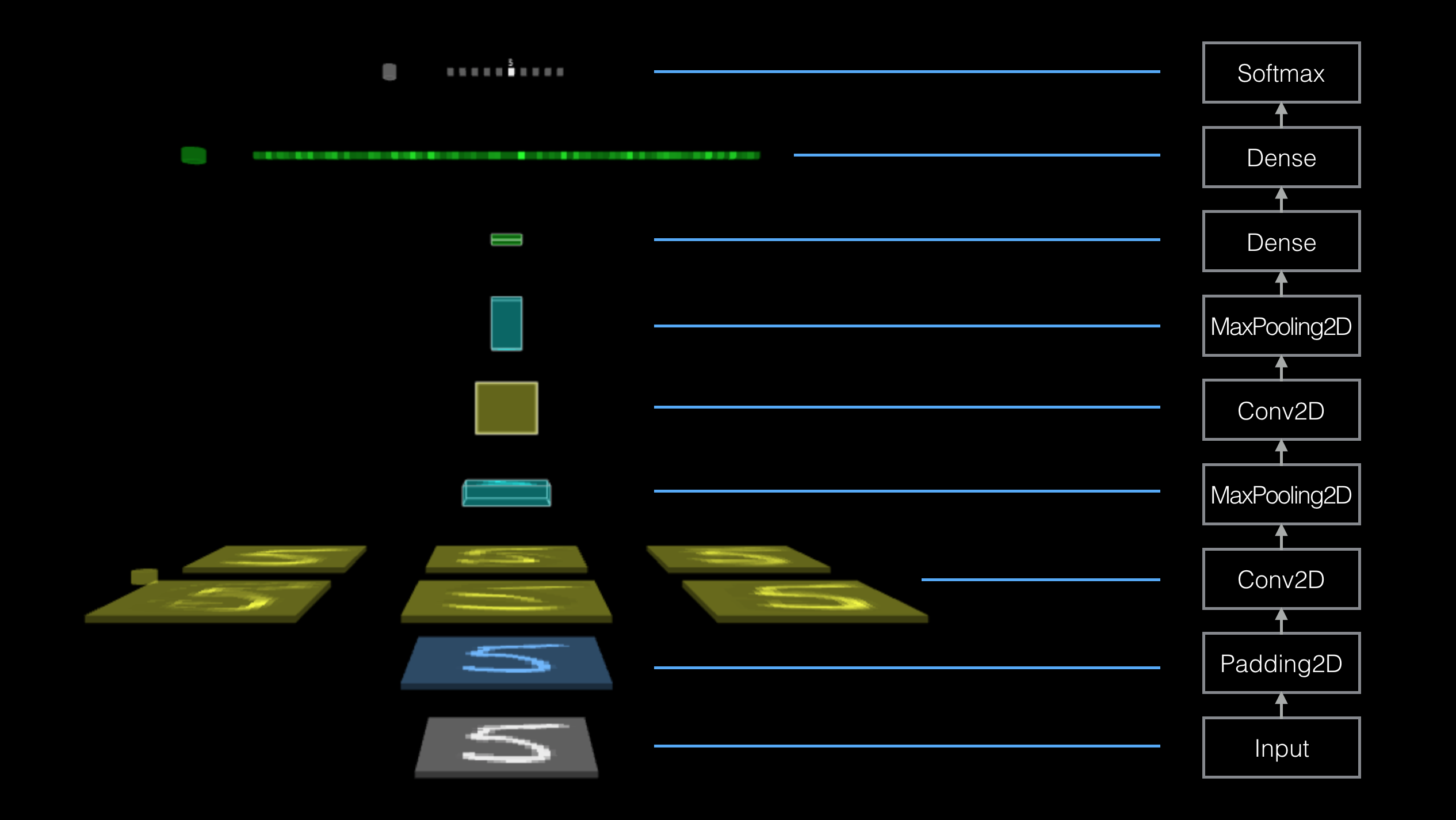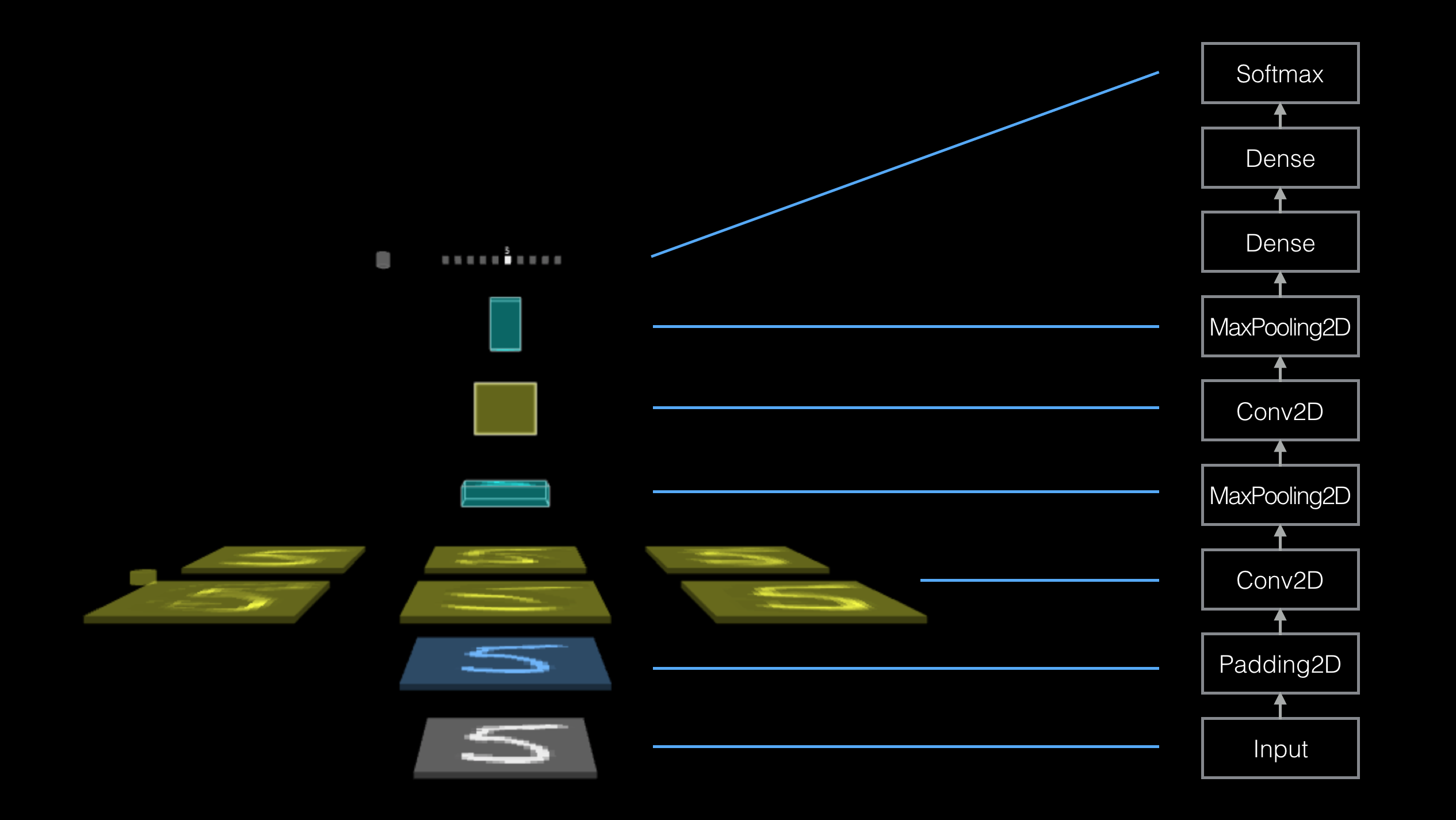TensorSpace.js
Getting Start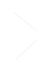Basic ConceptsTensorSpace ConverterModel PreprocessingModelsLayersMerge Function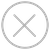Construct Topology
In TensorSpace, TensorSpace topology construction is the way to customize the 3D visualization which will reproduce the pre-trained network's topology. We use different ways to construct topology for TensorSpace Sequential Model and TensorSpace Functional Model.
Construct Sequential Model Topology
The TensorSpace Sequential model has the same concept as Keras's Sequential Model -- a linear stack of layers. In TensorSpace, we can use Sequential model's .add() function to add layers to model, and TensorSpace will automatically build a visualization stack topology in initialization process.
For example, if you want to have a following visualization sequential topology:Fig. 1 - Sequential Topology
Use the following code snip:
``````let model = new TSP.models.Sequential( modelContainer );

outputs: [ "0", "1", "2", "3", "4", "5", "6", "7", "8", "9" ]
} ) );

});

model.init();``````
Construct Functional Model Topology
The TensorSpace Functional model has the same concept as Keras's Functional Model -- functional model API can be used to define complex models. In TensorSpace, we can use Layer's .apply() API and Merge Function to make connection between Layers, and configure Model's inputs and outputs attribute to define functional model's input Layers and output Layers. TensorSpace will infer from Model's inputs, Model's outputs, connection between TensorSpace Layers to build a visualization topology in initialization process.
For example, if you want to have a following visualization functional topology: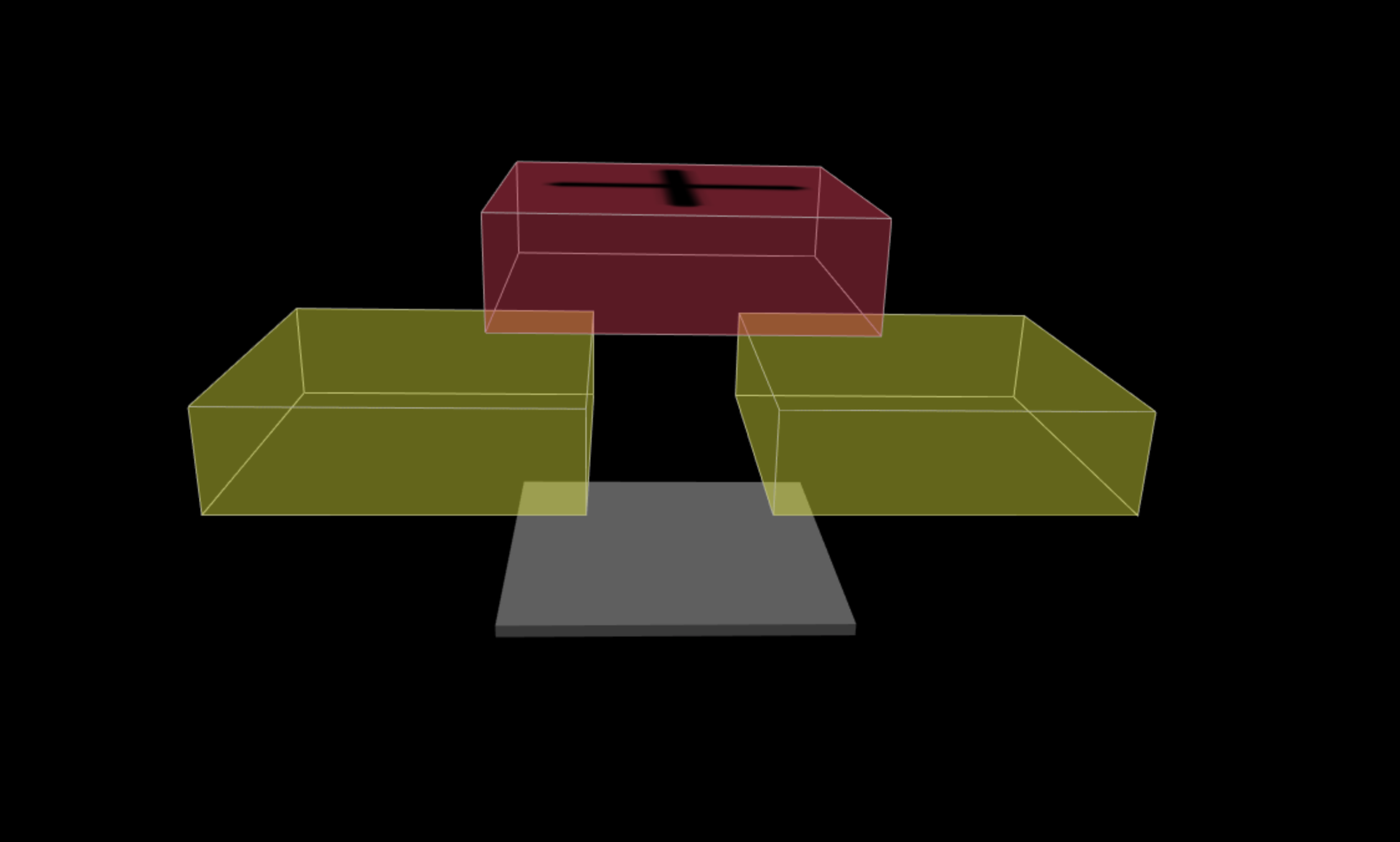Fig. 2 - Functional Topology
Use the following code snip:
``````let input = new TSP.layers.GreyscaleInput();

let conv2d_1 =  new TSP.layers.Conv2d();
conv2d_1.apply(input);

let conv2d_2 =  new TSP.layers.Conv2d();
conv2d_2.apply(input);

let model = new TSP.models.Model(modelContainer, {
inputs: [input],
});